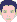## (6): Default Moisture Storage Function

support
WUFI UserPosts: 33
Joined: Thu Mar 03, 2005 2:35 am -1100

### (6): Default Moisture Storage Function

When I do not define a moisture storage function for a material, WUFI uses a default moisture storage function instead. What does this function look like?

WUFI needs a well-defined moisture field for each time step, so it must assign a moisture content even to materials which nominally don't have any appreciable moisture content (e.g. water-repellent mineral wool, air layers etc.).

The default moisture storage function used by WUFI is described by the function
w = a / (b – phi) + c
w : water content [kg/m³]
phi : relative humidity [-]

Since phi must be 0 for w = 0, it follows immediately that
c = -a/b

The constants a and b are determined as follows:
b is set to 1.0105.
The moisture content at free saturation, wf, corresponds to a relative humidity of 1 (=100%). Since WUFI also needs a unique relationship between moisture content and RH for moisture contents above free saturation, this oversaturation region is assigned RHs greater than 1, up to phimax = 1.01. This value phimax is reached when the moisture content reaches maximum saturation wmax which is determined by the porosity:
wmax = porosity * 1000 kg/m³

Therefore we have
wmax = a / (b-phimax) – a/b.
Solving for a yields:
a = wmax * b * (b – phimax) / phimax,
and thus:
w / wmax = phi / (b – phi) * (b – phimax) / phimax.

In particular, for phi = 1 we have
wf / wmax = 1 / (b – 1) * (b – phimax) / phimax = 0.047.
So this pseudo material has a free saturation of wf = 0.047 wmax.